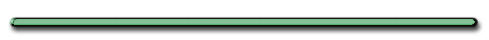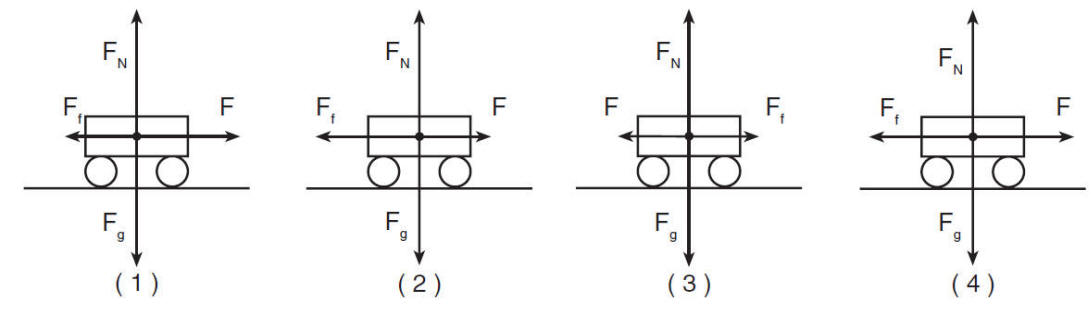"What values would a bathroom scale have on  a trip from the 1st to the fifteenth floor?"

Imagine a person weighs 600. NStart - Scale reading goes up
(greater than 600. N) Inertia
(Object rest tends to stay at rest)

Most of trip up:

(W = 600. N) Constant velocity
Net force = 0

Slow Down and Stop
- (Scale reading less than 600. N)
Object in motion tends to stay in motion

Is this elevator going up or down?

The elevator was going down.

2nd Law - Relationship between F, m, aInertia - Dr. Dave Hall of FameUnbalanced forces
cause objects to accelerate:

 (m) a = Fnet (N) (s2) m (Kg)

Fnet = Resultant1.  Acceleration is directly proportional to the force exerted on an objecta F x 3 x 3 x 2 x 2 divided by 3 divided by 3 divided by 2 divided by 2 x 2/3 x 2/3 quartered quartered"All cars are moving to the right. Which are speeding up. Which are slowing down? Which are moving at constant velocity"1 - Speeding up 2 - Slowing Down, 3,4 constant velocityCheck out this